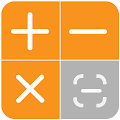# Calculator Plus APK

Calculator Plus is an easy-to-use photo calculator, scan to solve any math equation in no time! Not only can it do what scientific calculator and math calculator do like calculus calculation, trigonometric calculation, but it also acts as your GPA calculator, BMI calculator and even discount calculator! With its picture calculator scan capability, calculation has never been so easy and fun!All-in-one calculator, multi – functional
Powerful calculating functions packed into one single app, Calculator Plus is more than just a photo math calculator. It can calculate your GPA scores and BMI, just to name a few.

Photo calculator/picture calculator, easy and fun
Rigged with powerful photo math calculator technology, you can leave difficult math problems and math calculation to your camera. Scan to solve math equations with camera calculator scan built-in, calculus problems will be just as easy.

Currency calculator, accurate exchange rate
Apart from the powerful photo calculator, it can help you with money things. Using the updated currency exchange rates, you can simply type in the number and convert it into your chosen currency with Calculator Plus.

GPA calculator, keep track of your scores
Calculate your GPA score, GPA calculator can help you keep an eye on your GPA scores. Calculator Plus is more than just a math calculator or photo calculator app.

Scientific calculator, powerful calculating
With Calculator Plus, you can solve trigonometric and calculus problems, as well as complex math equations. Calculating has never been so easy.

A math calculator, a GPA calculator, Calculator Plus is many things in one. You don’t have to download too many apps, just use our smart calculator — Calculator Plus app and get all the powerful features like photo calculator scan, unit converter and camera calculator to name just a few.

If you come across one of those ads, please report to us by emailing to [email protected]
Please find our official policy here:
developertech.online/ca4/policy.html

#### What’s New

Take photo to solve the math problem with Calculator Plus.